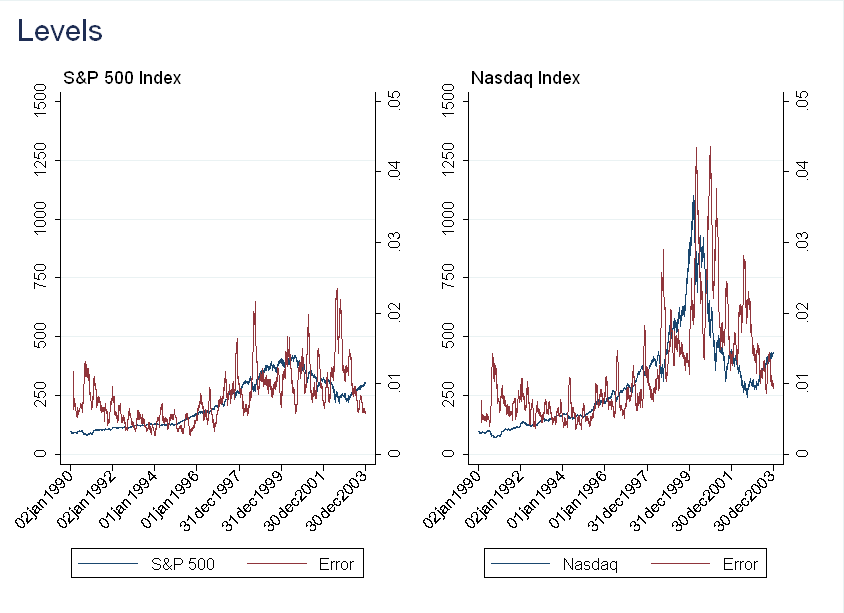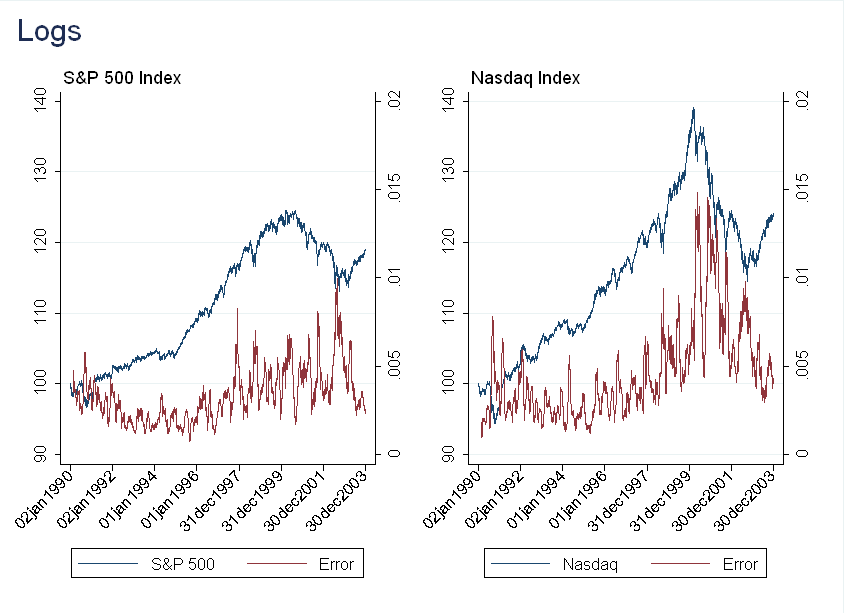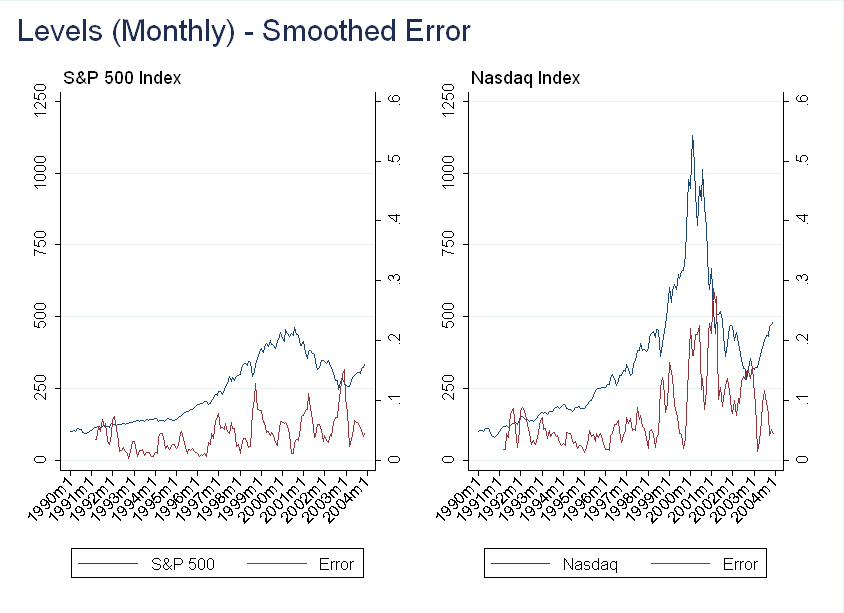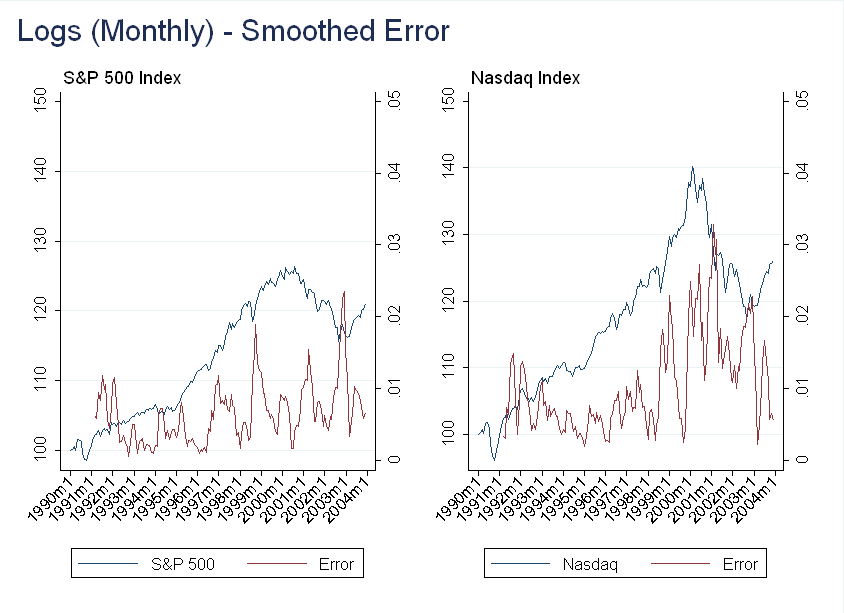This is the fifth post in a series on bubbles in the U.S. Equity Market. The first part can be found here.

# Overview

In Part 2, we discussed the test developed by Phillips et al. to detect bubbles. As I mentioned in that post, the ADF test is basically looking for extreme return persistence. This gave me an idea - in the presence of a “bubble”, returns should become more predictable. This posts sets up a simple model and tests it empirically with the Nasdaq “bubble” discussed in Part 3.

# Simple Model

Consider the classical asset pricing model, where dividends are the only fundamentals: \begin{equation} p_t = E_t \left [\sum\limits_{j=1}^\infty \beta^j \frac{u’(c_{t+j})}{u’(c_t)} d_{t+j} \right] + B_t \end{equation} Now, suppose the process for dividends follows a random walk without drift: \begin{equation} d_{t+1}=d_t + \epsilon_t \end{equation} where $\epsilon_t$ is white noise. Further, suppose the bubble term $B_t=0$. Under these assumptions, the stock price follows a random walk as well, so $E_t [p_{t+1}|\Omega_t]=p_t$. In other words, our best forecast of tomorrow’s price, $p_{t+1}$, is the price today, $p_t$.
Now suppose $B_t\neq0$ and $E_t(B_{t+1})=(1+r)B_t$. With dividends still following a random walk without drift, our best forecast of tomorrow’s price is $p_t + (1+r)B_t$. As long as the bubble persists, the bubble term should dominate the asset’s returns, and on average, the price should grow at rate $r$ (assuming the variance of $\epsilon$ is sufficiently small).
To convince you of this, consider a simplified model with linear utility. Then with no bubble, and using the fact that $E_t[d_{t+j}] = d_t$: \begin{equation} p_t=E_t \Big[\beta d_{t+1} + \beta^2 d_{t+2} + \dots \Big] = \frac{\beta}{1-\beta} d_t \end{equation} Applying the law of iterated expectations: \begin{equation} E_t[p_{t+1}]=E_t \Big[\beta d_{t+2} + \beta^2 d_{t+3} + \dots \Big] = \frac{\beta}{1-\beta} d_t=p_t \end{equation} Now suppose we have a bubble: \begin{equation} p_t=E_t \Big[\beta d_{t+1} + \beta^2 d_{t+2} + \dots \Big] + B_t= \frac{\beta}{1-\beta} d_t + B_t \end{equation} Again applying the law of iterated expectations: \begin{equation} E_t[p_{t+1}]=E_t \Big[\beta d_{t+2} + \beta^2 d_{t+3} + \dots \Big] + E_t[B_{t+1}] = \frac{\beta}{1-\beta} d_t +(1+r)B_t=p_t+(1+r)B_t \end{equation} Let $TR_i$ denote the total return of an asset over the past $i$ periods. Then, in the presence of a bubble: \begin{equation} (TR_i+1)^{\frac{1}{6}}-1 =\hat{r} \approx r \end{equation} To test for a bubble, construct two forecasts:
1) Under no bubble: $\hat{p}_{t+1}=p_t$
2) With a bubble: $\hat{p}_{t+1}=p_t(1+\hat{r})$
Finally, (mis)use the Diebold-Mariano test to compare the forecasts (I know this is not the intended use for the Diebold-Mariano test - this will be the topic of a future blog post when I review Diebold (2013)).

# Relative Forecast Error

Before getting into the test described above, I wanted to make a first pass with some “eyeball econometrics” (a great quote from Uhlig (2005)). Note - I present all my results here, not just the ones that worked the way I wanted. Only reporting positive results and data snooping is a much bigger issue, and it will be the topic of a future blog post.

# Daily Data

For now, forget the model. Suppose we only use the idea that returns become more predictable in a bubble. Using daily data run the following regression:
$r_t = \hat{\beta} y_{t-1} + \hat{\gamma}_1 r_{t-1} + \dots + \hat{\gamma}_p r_{t-p} + \epsilon_t$
where $r$ is returns and $y$ is the level (for example - the value of the Nasdaq or S&P 500 index). Use this to forecast $\hat{r}_t$, and then forecast $\hat{y_t}=y_{t-1}(1+\hat{r}_t)$.
The exact procedure I used is:
1) Select $p=2$ based on AIC and BIC 2) Use a 30 day rolling window to run the regression and calculate a one-step-ahead forecast
3) Compute the relative forecast error as $\frac{|y_t-\hat{y}_t|}{y_t}$
4) Take a 30 day moving average of forecast error to remove noise
In the figure below, the first observation for $y_t$ is normalized to 100.The result here is counterintuitive. Although we think returns become more predictable during a bubble, the forecasting actually gets worse! A possible explanation is that in a bubble, growth is exponential, rather than linear, so I repeated the exercise with $y_t'=ln(y_t)$. The results are very similar.Given these two failures, I was worried that the data might be too noisy at the daily frequency to get good predictions (recall in Part 3 I discussed how the volatility of the index was very high at the time). The next section repeats the exercise with monthly data.

# Monthly Data

The plot below is computed using the same methodology as above, except I did not smooth the error:You can see there is still a large relative error during the bubble, but it is also very volatile. Smoothing the error at the quarterly frequency (using two prior months and current month) we get:Now, this is interesting - we get a dip in the forecasting error in the middle of 1999. I don’t think it’s worth reading into this too much (as I had to do several transformations before finding this result), but there may be something here.

# Forecasting Performance

Given the results above, it doesn’t seem like returns will be much more predictable during a bubble. That being said, I still think it is a worthwhile exercise to test the model described above.
The procedure I used to calculate forecasts is as follows:
1) Use 6 months of data to compute $\hat{r}$
2) Compute two forecasts, one being $\hat{y}_t^1=y_{t-1}$ and $\hat{y}_t^2=y_{t-1}(1+\hat{r})$
3) Use the Diebold-Mariano test to compare the two forecasts
Doing this for 1998-2001 (inclusive), the random walk is better (at the 5% level) for forecasting the S&P 500 than the bubble model. For the Nasdaq, the forecasts are not significantly different at the 5% level (although the random walk model is better at the 10% level).
As above, there is not much of a difference, but there is a difference. I don’t think it makes sense to go too much further down this route without better theoretical justification, as at some point we are just data snooping.

# Conclusion

Although the results were not what I expected, they are unsurprising. During a bubble we don’t just have high returns, but volatile returns as well. Even if returns become more predictable, this is obscured by high volatility.